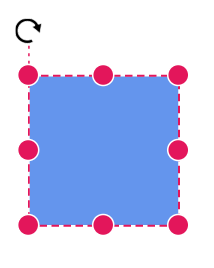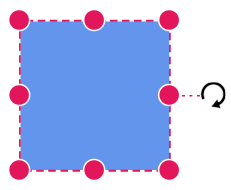# Positioning a node in Blazor Diagram Component

2 Feb 20227 minutes to read

## Arrange the nodes

• Position of a node is controlled by using the OffsetX and OffsetY properties. By default, these offset properties represent the distance between the origin of the diagram’s page and node’s center point.

• You may expect this offset values to represent the distance between page origin and node’s top-left corner instead of center. The Pivot property helps to solve this problem. Default value of node’s Pivot point is (0.5, 0.5) that means center of the node.

• The size of the node can be controlled by using the Width and Height properties.

The following table shows how pivot relates offset values with node boundaries.

Pivot Offset
(0.5,0.5) OffsetX and OffsetY values are considered as the node’s center point.
(0,0) OffsetX and OffsetY values are considered as the top-left corner of the node.
(1,1) OffsetX and OffsetY values are considered as the bottom-right corner of the node.

The following code shows how to change the Pivot value.

``````@using Syncfusion.Blazor.Diagram
@using System.Collections.ObjectModel

<SfDiagramComponent Height="600px" @ref="@diagram" Nodes="@nodes" />

@code
{
SfDiagramComponent diagram;
DiagramObjectCollection<Node> nodes;

protected override void OnInitialized()
{
nodes = new DiagramObjectCollection<Node>();
// A node is created and stored in nodes array.
Node node = new Node()
{
ID = "node",
// Position of the node.
OffsetX = 250,
OffsetY = 250,
// Size of the node.
Width = 100,
Height = 100,
Style = new ShapeStyle()
{
Fill = "#6495ED",
StrokeColor = "white"
},
// Pivot of the node.
Pivot = new DiagramPoint() { X = 0, Y = 0 }
};
}

protected override async Task OnAfterRenderAsync(bool firstRender)
{
if (firstRender)
{
//OnAfterRenderAsync will be triggered after the component rendered.
diagram.Select(new ObservableCollection<IDiagramObject>() { diagram.Nodes });
}
}
}``````Rotation of a node is controlled by using the RotationAngle property. The following code shows how to change the RotationAngle value.

``````@using Syncfusion.Blazor.Diagram

<SfDiagramComponent Height="600px" @ref="@diagram" Nodes="@nodes" />

@code
{
SfDiagramComponent diagram;
DiagramObjectCollection<Node> nodes;

protected override void OnInitialized()
{
nodes = new DiagramObjectCollection<Node>();
// A node is created and stored in nodes array.
Node node = new Node()
{
ID = "node",
// Position of the node.
OffsetX = 250,
OffsetY = 250,
// Size of the node.
Width = 100,
Height = 100,
Style = new ShapeStyle()
{
Fill = "#6495ED",
StrokeColor = "white"
},
// RotationAngle of the node.
RotationAngle = 90
};
}
}``````## How to set minimum size and maximum size for the node

The MinWidth and MinHeight properties of node allows you to control the minimum size of the node while resizing. Similarly, the MaxWidth and MaxHeight properties of node allows you to control the maximum size of the node while resizing. The below gif explains how minimum and maximum size is controlled.

``````@using Syncfusion.Blazor.Diagram

<SfDiagramComponent Height="600px" @ref="@diagram" Nodes="@nodes" />

@code
{
SfDiagramComponent diagram;
DiagramObjectCollection<Node> nodes;

protected override void OnInitialized()
{
nodes = new DiagramObjectCollection<Node>();
// A node is created and stored in nodes array.
Node node = new Node()
{
ID = "node",
// Position of the node.
OffsetX = 250,
OffsetY = 250,
// Size of the node.
Width = 100,
Height = 100,
//Minimum Size of the node.
MinHeight = 50,
MinWidth = 50,
//Maximum Size of the node.
MaxHeight = 200,
MaxWidth = 200,
Style = new ShapeStyle()
{
Fill = "#6495ED",
StrokeColor = "white"
},
};